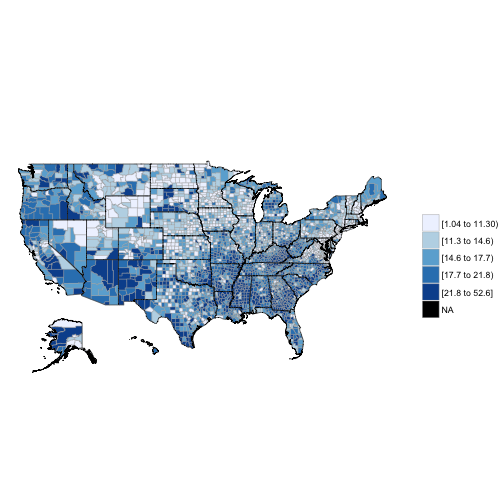The acsr package helps extracting variables and computing statistics using the America Community Survey and Decennial US Census. It was created for the Applied Population Laboratory (APL) at the University of Wisconsin-Madison.

## Installation

The functions depend on the acs and data.table packages, so it is necessary to install then before using acsr. The acsr package is hosted on a github repository and can be installed using devtools:

Remember to set the ACS API key, to check the help documentation and the default values of the acsr functions.

The default dataset is acs, the level is state (Wisconsin, state = "WI"), the endyear is 2014, and the confidence level to compute margins of error (MOEs) is 90%.

## Levels

The acsr functions can extract all the levels available in the acs package. The table below shows the summary and required levels when using the acsdata and sumacs functions:

summary number levels
010 us
020 region
030 division
040 state
050 state, county
060 state, county, county.subdivision
140 state, county, tract
150 state, county, tract, block.group
160 state, place
250 american.indian.area
320 state, msa
340 state, csa
350 necta
400 urban.area
500 state, congressional.district
610 state, state.legislative.district.upper
620 state, state.legislative.district.lower
795 state, puma
860 zip.code
950 state, school.district.elementary
960 state, school.district.secondary
970 state, school.district.unified

## Getting variables and statistics

We can use the sumacs function to extract variable and statistics. We have to specify the corresponding method (e.g., proportion or just variable), and the name of the statistic or variable to be included in the output.

To download the data can be slow, especially when many levels are being used (e.g., blockgroup). A better approach in those cases is, first, download the data using the function acsdata , and then use them as input.

## Standard errors

When computing statistics there are two ways to define the standard errors:

• Including all standard errors of the variables used to compute a statistic (one.zero = FALSE)
• Include all standard errors except those of variables that are equal to zero. Only the maximum standard error of the variables equal to zero is included (one.zero = TRUE)
• The default value is one.zero = TRUE

For more details about how standard errors are computed for proportions, ratios and aggregations look at A Compass for Understanding and Using American Community Survey Data.

Below an example when estimating proportions and using one.zero = FALSE:

$SE = \sqrt{ \frac{(5.47 ^ 2 + 22.49 ^ 2 + 5.47 ^ 2) - ( 0.02 ^ 2 \times 102.13 ^ 2)}{1546} } \times 1.645 = 0.0252$

When one.zero = TRUE:

$SE_{\text{ one.zero}} \sqrt{ \frac{(5.47 ^ 2 + 22.49 ^ 2) - ( 0.02 ^ 2 \times 102.13 ^ 2)}{1546} } \times 1.645 = 0.0245$

When the square root value in the standard error formula doesn’t exist (e.g., the square root of a negative number), the ratio formula is instead used. The ratio adjustment is done variable by variable .

It can also be that the one.zero option makes the square root undefinable. In those cases, the function uses again the ratio formula to compute standard errors. There is also a possibility that the standard error estimates using the ratio formula are higher than the proportion estimates without the one.zero option.

## Decennial Data from the US Census

Let’s get the African American and Hispanic population by state. In this case, we don’t have any estimation of margin of error.

## Output

The output can be formatted using a wide or long format:

And it can also be exported to a csv file:

## Combining geographic levels

We can combine geographic levels using two methods: (1) sumacs and (2) combine.output. The first one allows only single combinations, the second multiple ones.

If I want to combine two states (e.g., Wisconsin and Minnesota) I can use:

If I want to put together multiple combinations (e.g., groups of states):

## A map?

Let’s color a map using poverty by county:In sum, the acsr package:

• Reads formulas directly and extracts any ACS/Census variable
• Provides an automatized and tailored way to obtain indicators and MOEs
• Allows different outputs’ formats (wide and long, csv)
• Provides an easy way to adjust MOEs to different confidence levels
• Includes a variable-by-variable ratio adjustment of standard errors
• Includes the zero-option when computing standard errors for proportions, ratios, and aggregations
• Combines geographic levels flexibly

Last Update: 02/07/2016